# Python data manipulation from Pandas LibraryThe Pandas library is the most popular Python data manipulation library. It provides an easy way to manipulate data through its data-frame API, inspired from R’s data-frames.

The Pandas library is the most popular Python data manipulation library. It provides an easy way to manipulate data through its data-frame API, inspired from R’s data-frames.

## Understanding The Pandas Library

One of the keys to getting a good understanding of Pandas, is to understand that Pandas is mostly a wrapper around a series of other Python Libraries. The main ones being Numpy, SQL Alchemy, Matplot lib and Openpyxl.

The core internal model of the data-frame is a series of Numpy array’s, and Pandas functions such as the now deprecated “as_matrix” return results in that internal representation.

Pandas leverages other libraries to get data in and out of data-frames, SQL Alchemy for instances is used through the read_sql and to_sql functions. And openpyxl and xlsx writer are used for read_excel and to_excel functions.

Matplotlib and Seaborn are instead used to provide an easy interface to plot information available within a data frame, using command such as df.plot()

## Numpy’s Panda — Efficient pandas

One of the complain that you often hear is that Python is slow or that it is difficult to handle large amount of data. Most often than not, this is due to poor efficiency of the code being written. It is true that native Python code tends to be slower than compiled code, but libraries like Pandas effectively provides an interface in Python code to compiled code. Knowing how to properly interface with it, let us get the best out of Pandas/Python.

#### APPLY & VECTORIZED OPERATIONS

Pandas, like its underlying library Numpy, performs vectorized operations more efficiently than performing loops. These efficiencies are due to vectorized operations being performed through C compiled code, rather than native python code and on the ability of vectorized operations to operate on entire datasets.

The apply interface allows to gain some of the efficiency by using a CPython interfaces to do the looping:

``````df.apply(lambda x: x['col_a'] * x['col_b'], axis=1)
``````

But most of the performance gain would be obtained from the use of vectorized operation themselves, be it directly in pandas or by calling its’ internal Numpy arrays directly.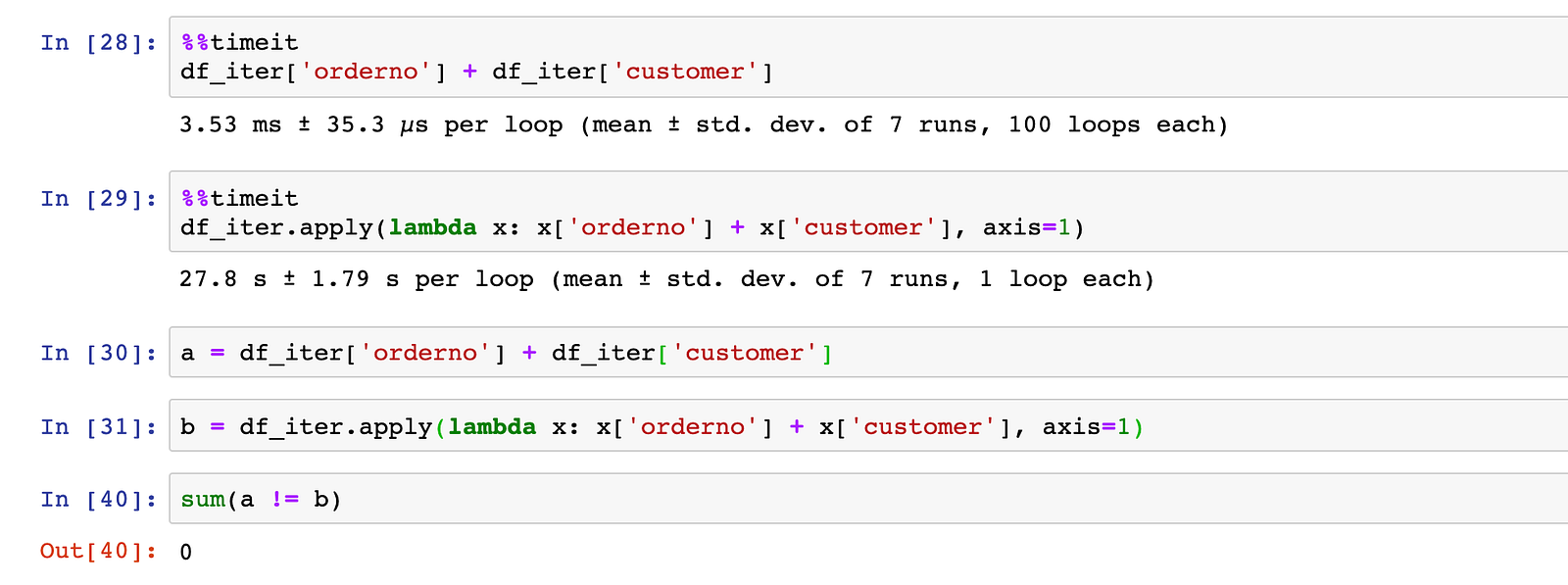As you can see from the picture above the difference in performance can be drastic, between processing it with a vectorized operation (3.53ms) and looping with apply to do an addition (27.8s). Additional efficiencies can be obtained by directly invoking the numpy’s arrays and api, eg: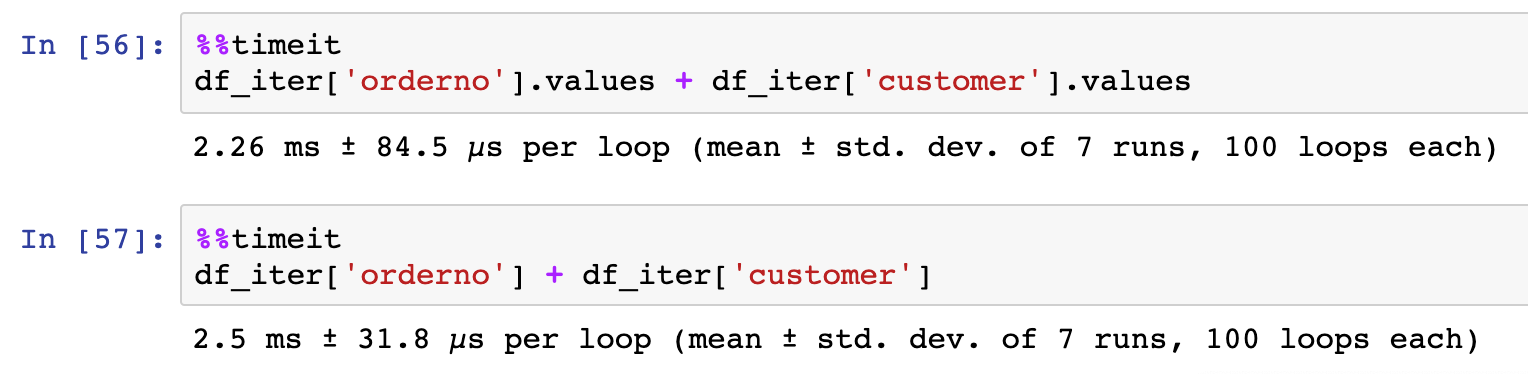*Swifter: *swifter is a python library that makes it easy to vectorize different types of operations on dataframe, its API is fairly similar to that of the apply function

#### EFFICIENT DATA STORING THROUGH DTYPES

When loading a data-frame into memory, be it through read_csv, or read_excel or some other data-frame read function, SQL makes type inference which might prove to be inefficient. These api allow you to specify the types of each columns explicitly. This allows for a more efficient storage of data in memory.

``````df.astype({'testColumn': str, 'testCountCol': float})
``````

Dtypes are native object from Numpy, which allows you to define the exact type and number of bits used to store certain informations.

Numpy’s dtype `np.dtype('int32')` would for instance represent a 32 bits long integer. Pandas default to set up integer to 64 bits, we could be save half the space when using a 32 bits: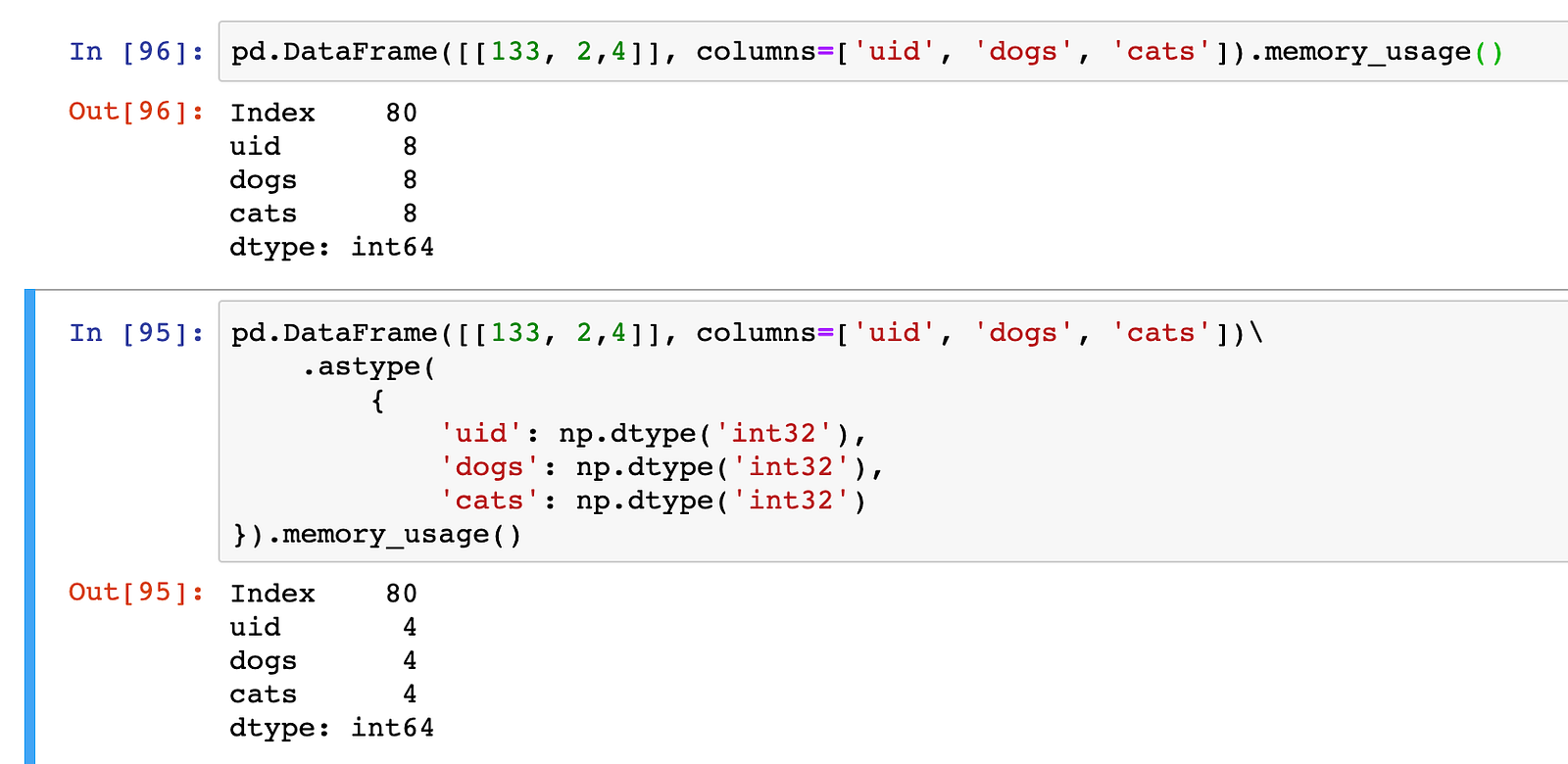memory_usage() shows the number of bytes used by each of the columns, since there is only one entry (row) per column, the size of each int64 column is 8bytes and of int32 4bytes.

Pandas also introduces the categorical dtype, that allows for efficient memory utilization for frequently occurring values. In the example below, we can see a 28x decrease in memory utilization for the field posting_date when we converted it to a categorical value.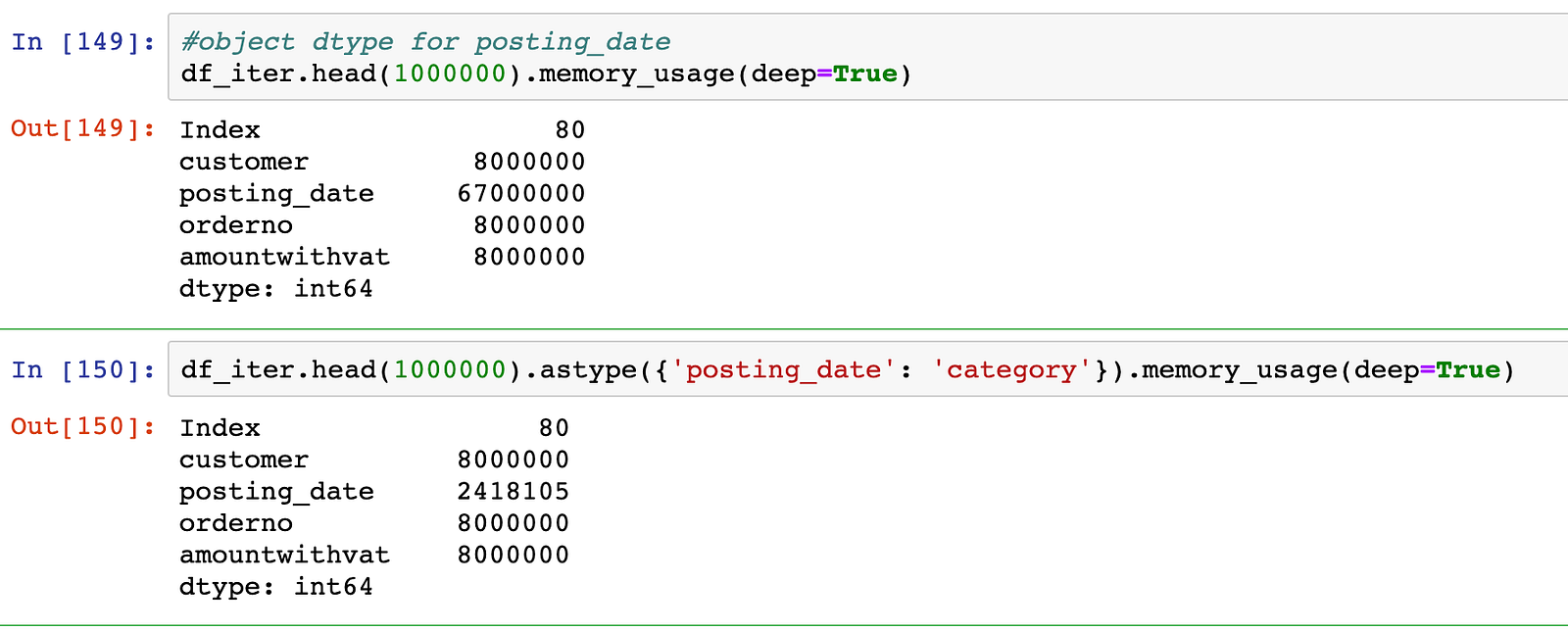In our example, the overall size of the data-frame drops by more than 3X by just changing this data type: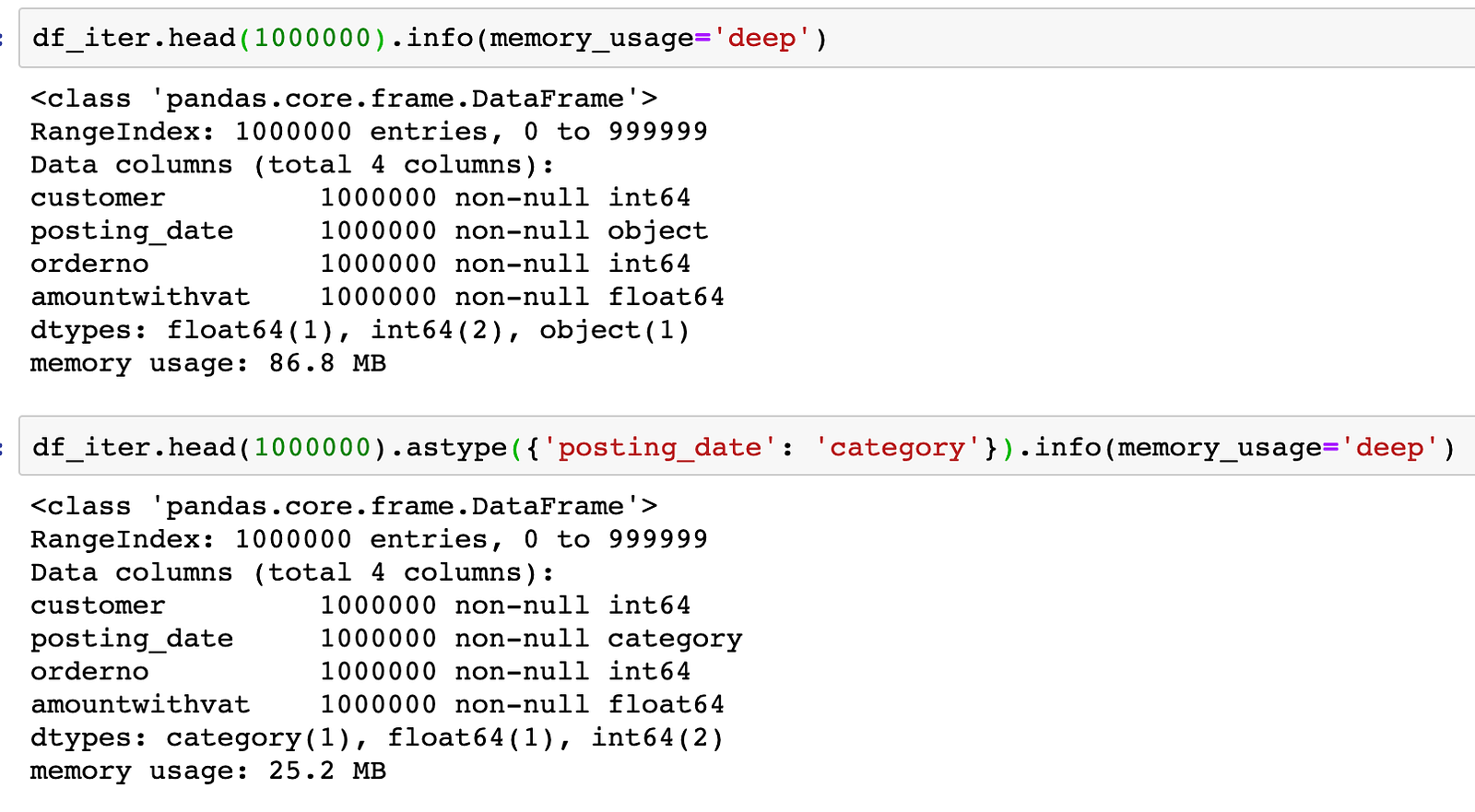Not only using the right dtypes allows you to handle larger datasets in memory, it also makes some computations become more effective. In the example below, we can see that using categorical type brought a 3X speed improvement for the groupby / sum operation.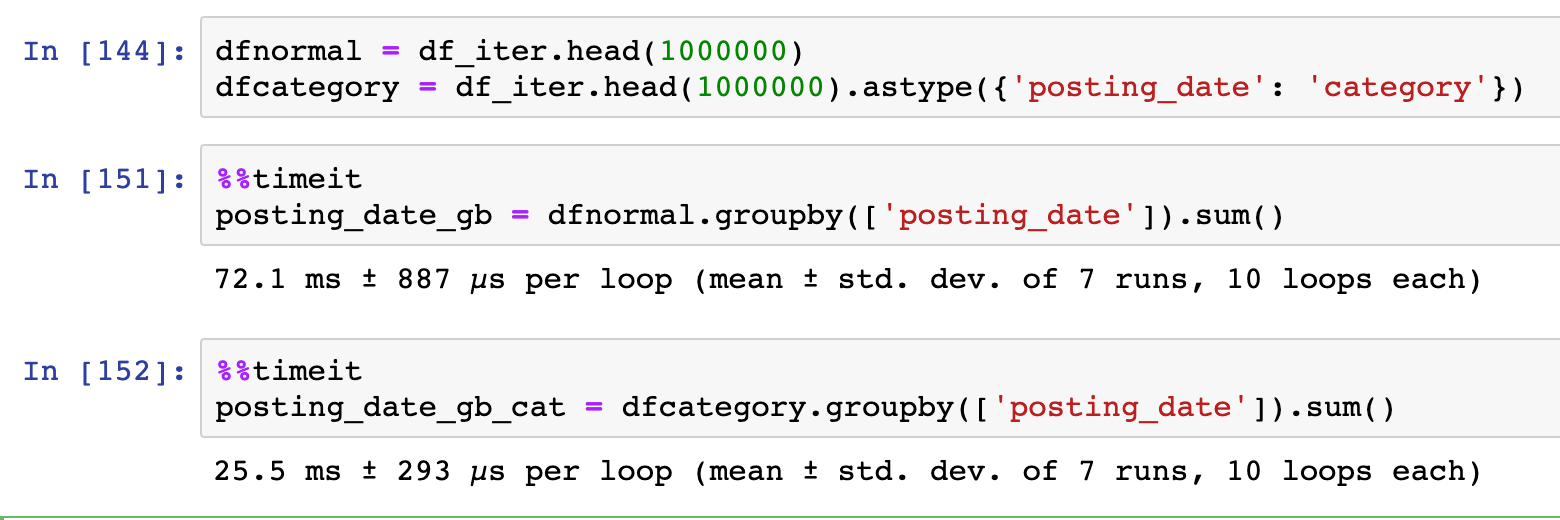Within pandas, you can define the dtypes during the data load (read_ ) or as a type conversion (astype).

*CyberPandas: *Cyber pandas is one of the different library extension that enables a richer variety of datatypes by supporting ipv4 and ipv6 data types and storing them efficiently.

#### HANDLING LARGE DATASETS WITH CHUNKS

Pandas allows for the loading of data in a data-frame by chunks, it is therefore possible to process data-frames as iterators and be able to handle data-frames larger than the available memory.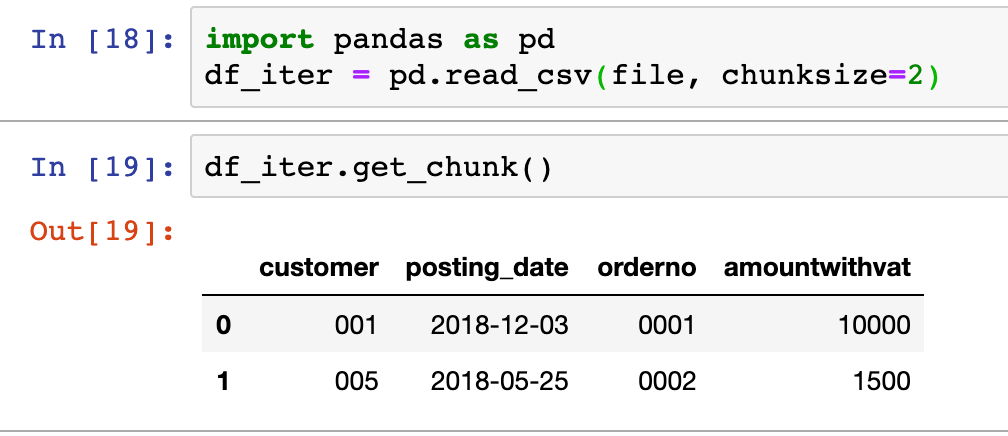The combination of defining a chunksize when reading a data source and the get_chunk method, allows pandas to process data as an iterator. For instance, in the example shown above, the data frame is read 2 rows at the time. These chunks can then be iterated through:

``````i = 0
for a in df_iter:
# do some processing  chunk = df_iter.get_chunk()
i += 1
new_chunk = chunk.apply(lambda x: do_something(x), axis=1)
new_chunk.to_csv("chunk_output_%i.csv" % i )
``````

The output of which can then be fed to a csv file, pickled, exported to a database, etc…

setting up operator by chunks also allows certain operations to be perform through multi-processing.

Dask: is a for instance, a framework built on top of Pandas and build with multi-processing and distributed processing in mind. It makes use of collections of chunks of pandas data-frames both in memory and on disk.

## SQL Alchemy’s Pandas — Database Pandas

Pandas also is built up on top of SQL Alchemy to interface with databases, as such it is able to download datasets from diverse SQL type of databases as well as push records to it. Using the SQL Alchemy interface rather than the Pandas’ API directly allows us to do certain operations not natively supported within pandas such as transactions or upserts:

#### SQL TRANSACTIONS

Pandas can also make use of SQL transactions, handling commits and rollbacks. Pedro Capelastegui, explained in one of his blog post notably, how pandas could take advantage of transactions through a SQL alchemy context manager.

``````with engine.begin() as conn:
df.to_sql(
tableName,
con=conn,
...
)
``````

the advantage of using a SQL transaction, is the fact that the transaction would roll back should the data load fail.

#### SQL extension

PandaSQL

Pandas has a few SQL extension such as pandasql a library that allows to perform SQL queries on top of data-frames. Through pandasql the data-frame object can be queried directly as if they were database tables.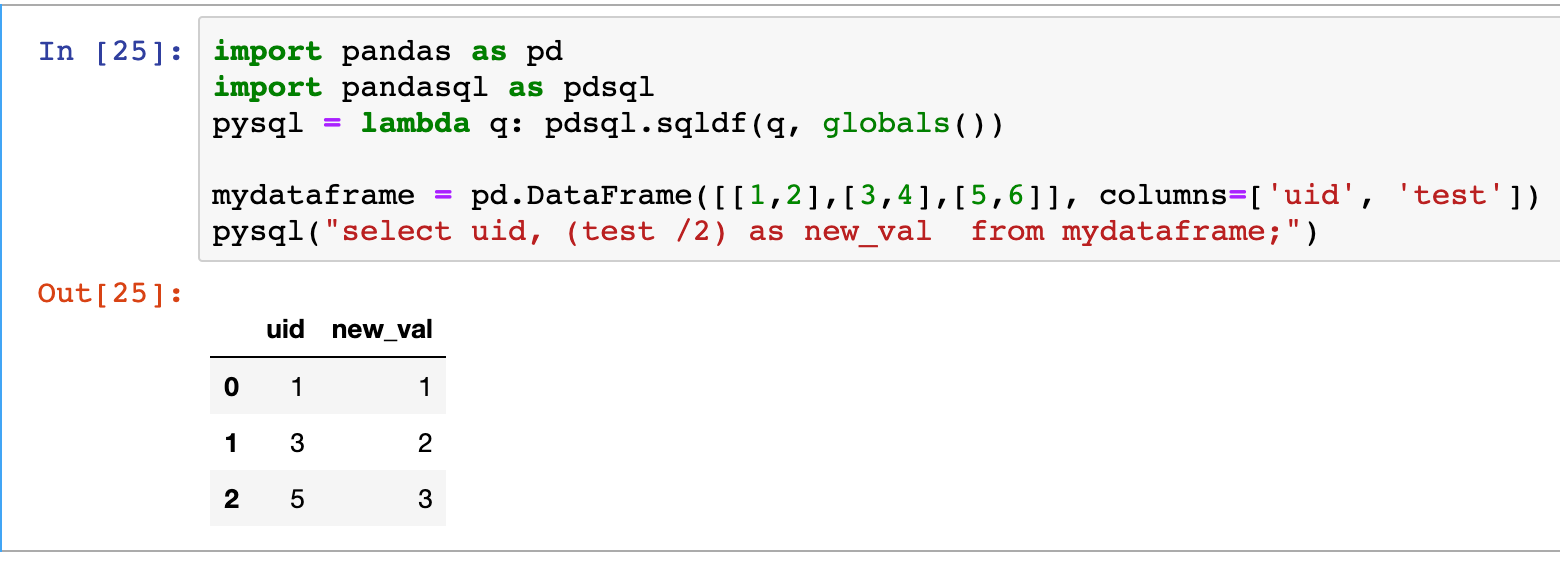SQL UPSERTs

Pandas doesn’t natively support upsert exports to SQL on databases supporting this function. Patches to pandas exists to allow this feature.

## MatplotLib/Seaborn — Visual Pandas

Matplotlib and Searborn visualization are already integrated in some of the dataframe API such as through the .plot command. There is a fairly comprehensive documentation as how the interface works, on pandas’ website.

Extensions: Different extensions exists such as Bokeh and plotly to provide interactive visualization within Jupyter notebooks, while it is also possible to extend matplotlib to handle 3D graphs.

### Other Extensions

Quite a few other extensions for pandas exists, which are there to handle no-core functionalities. One of them is tqdm, which provides a progress bar functionality for certain operations, another is pretty pandas which allows to format dataframes and add summary informations.

tqdm

tqdm is a progress bar extension in python that interacts with pandas, it allows user to see the progress of maps and applys operations on pandas dataframe when using the relevant function (progress_map and progress_apply):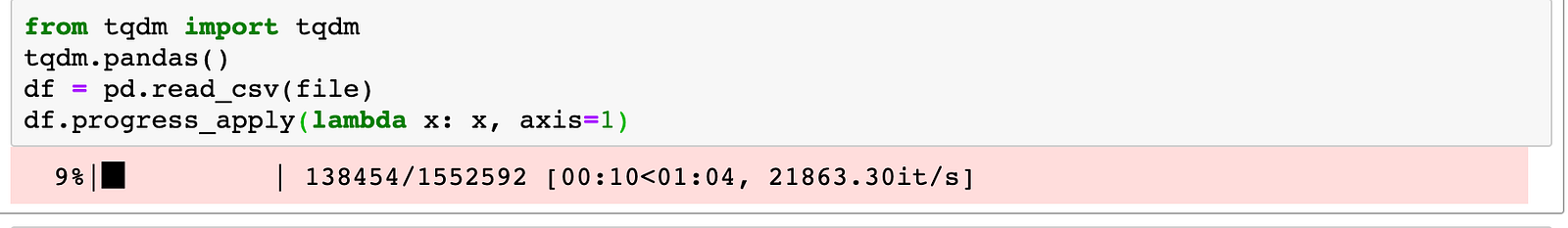PrettyPandas

PrettyPandas is a library that provides an easy way to format data-frames and to add table summaries to them: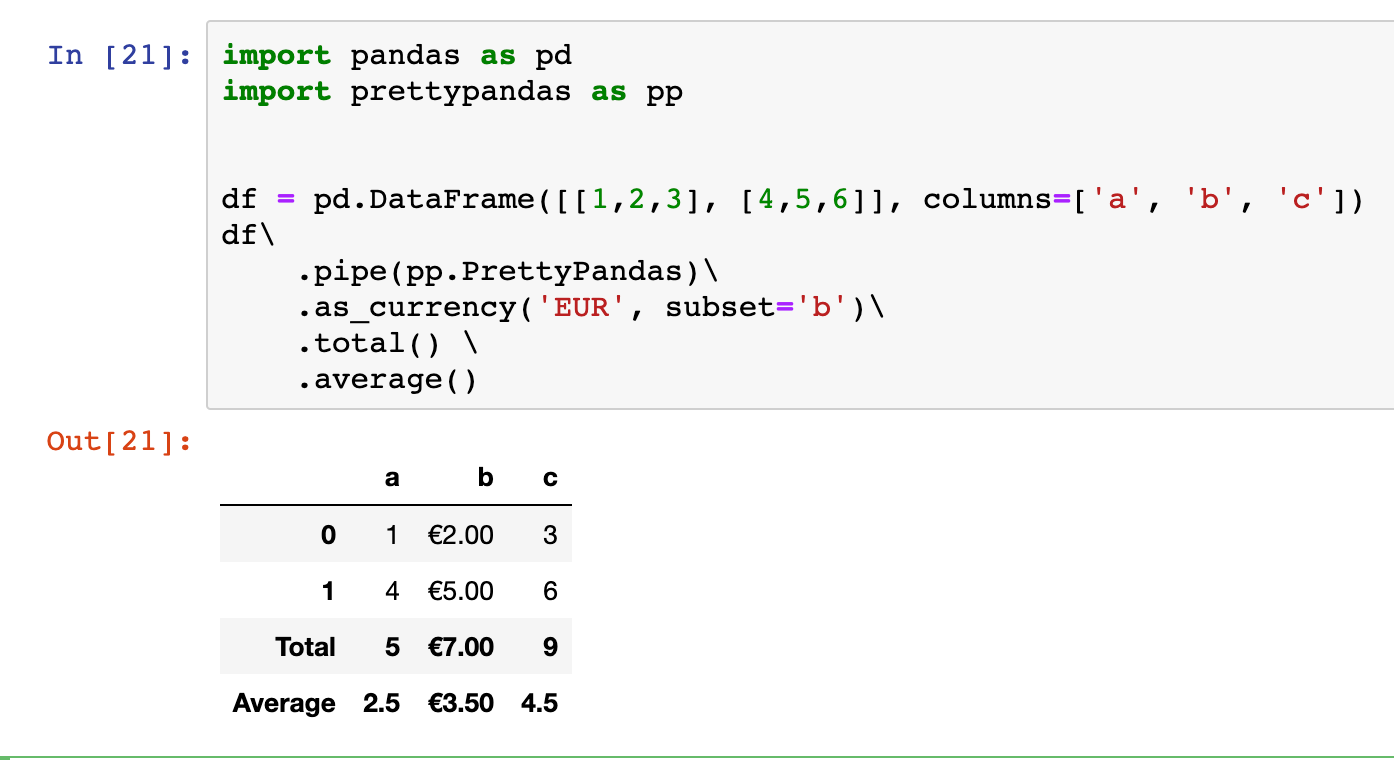## Python Pandas Tutorial - Data Analysis with Python Pandas

Python Pandas Tutorial will help you get started with Python Pandas Library for various applications including Data analysis. Introduction to Pandas. DataFrames and Series. How To View Data? Selecting Data. Handling Missing Data. Pandas Operations. Merge, Group, Reshape Data. Time Series And Categoricals. Plotting Using Pandas

## Data Science Course in Dallas

Become a data analysis expert using the R programming language in this [data science](https://360digitmg.com/usa/data-science-using-python-and-r-programming-in-dallas "data science") certification training in Dallas, TX. You will master data...

## Python For Data Science - How to use Data Science with Python

This Edureka video on 'Python For Data Science - How to use Data Science with Python - Data Science using Python ' will help you understand how we can use python for data science along with various use cases. What is Data Science? Why Python? Python Libraries For Data Science. Roadmap To Data Science With Python. Data Science Jobs and Salary Trends